GeeksforGeeks App
Open AppBrowser
Continue

# Shiny Package in R Programming

The package is an appropriate way to organize the work and share it with others. Packages in the R Programming language are a collection of R functions, compiled code, and sample data. They are stored under a directory called “library” in the R environment. By default, R installs a set of packages during installation. One of the most important packages in R is the Shiny package. Shiny is an R package that makes it easy to build interactive web apps straight from R. It helps to host standalone apps on a webpage or embed them in R Markdown documents or build dashboards. One can also extend Shiny apps with CSS themes, htmlwidgets, and JavaScript actions.

## Shiny package Install in R language

To use a package in R programming one must have to install the package first. This task can be done using the command install.packages(“packagename”). To install the whole Shiny package type this:

`install.packages("shiny")`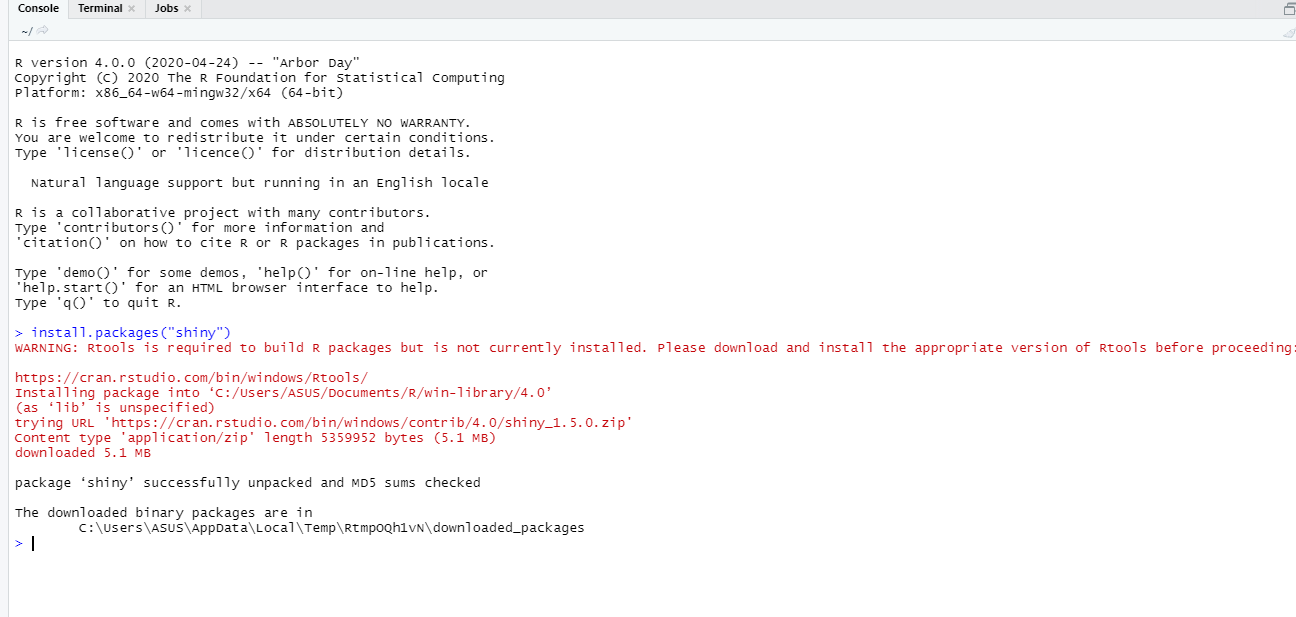To install the latest development builds directly from GitHub, run this instead:

```if (!require("remotes"))
install.packages("remotes")
remotes::install_github("rstudio/shiny")```

## shiny package in R Language Example

### Important Verb Functions in Shiny Package

• fluidPage(): It creates a page with a fluid layout. A fluid page layout consists of rows which in turn include columns. Rows exist for the purpose of making sure their elements appear on the same line and columns exist for the purpose of defining how much horizontal space within a 12-unit wide grid. Fluid pages scale their components in real-time to fill all available browser width.

Syntax:

fluidPage(…, title = NULL, theme = NULL)

Example:

This is a basic shiny app template.

## R

 `# import shiny package``library``(shiny)` `# define a page with fluid layout``ui <- ``fluidPage``(``h1``(``"GeeksforGeeks article on shiny package in R"``),``                ``p``(style = ``"font-family:Impact"``, ``"My first shiny app"``)``)``server <- ``function``(input, output) {}` `shinyApp``(ui = ui, server = server)`

Output: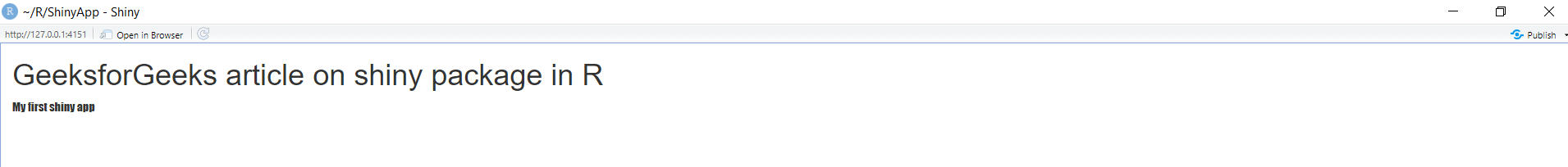• shinyApp(): It creates Shiny app objects from either an explicit UI/server pair or bypassing the path of a directory that contains a Shiny app.

Syntax:

shinyApp(ui, server, onStart = NULL, options = list(), uiPattern = “/”, enableBookmarking = NULL)

shinyAppDir(appDir, options = list())

shinyAppFile(appFile, options = list())</p

Example:

## R

 `# import shiny package``library``(shiny)` `# define fluid page layout``ui <- ``fluidPage``(``  ``sliderInput``(inputId = ``"num"``,``              ``label = ``"Choose a number"``,``              ``value = 10, min = 1, max = 1000),``  ``plotOutput``(``"hist"``)``)` `server <- ``function``(input, output)``{``  ``output\$hist <- ``renderPlot``({``    ``hist``(``rnorm``(input\$num))``  ``})``}` `# create shiny app object``# using shinyApp``shinyApp``(ui = ui, server = server)`

Output: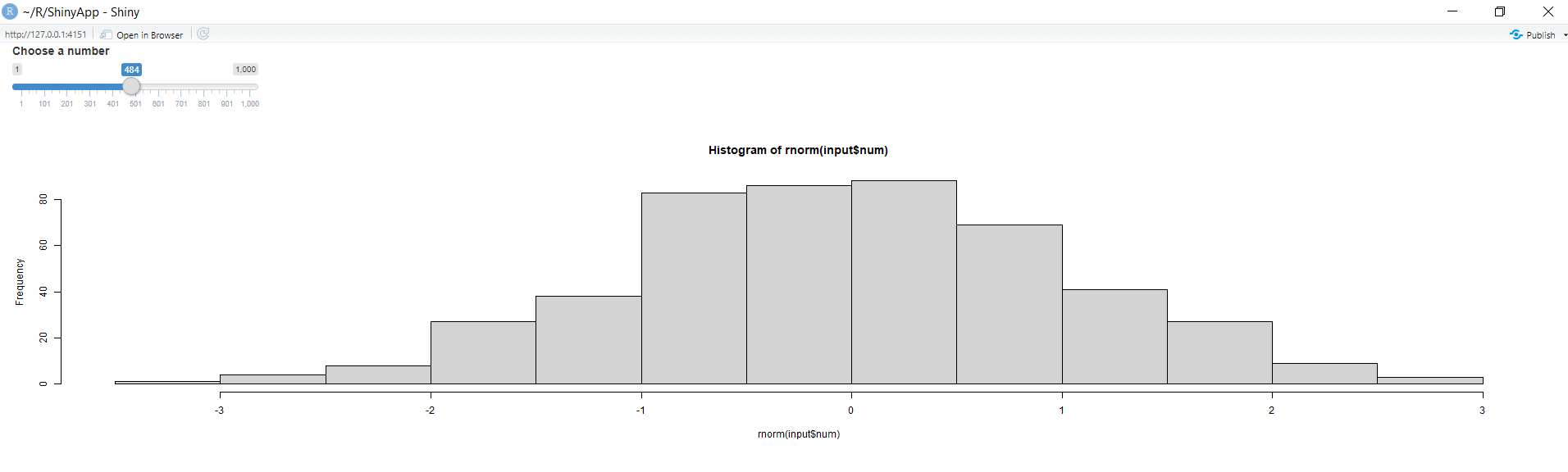• reactive(): It creates a reactive expression. A reactive expression is an expression whose result will change over time.reactive() wraps a normal expression to create a reactive expression.

Syntax: reactive(x, env = parent.frame(), quoted = FALSE, label = NULL)

Example:

## R

 `# import shiny package``library``(shiny)` `# define fluid page layout``ui <- ``fluidPage``(``  ``textInput``(inputId = ``"num"``, label = ``"Choose a number"``,``            ``value = ``""``, width = 100, placeholder = ``NULL``),``  ``plotOutput``(``"hist"``),``  ``verbatimTextOutput``(``"stats"``)``)``server <- ``function``(input, output)``{``  ``# use reactive to create``  ``# a reactive expression``  ``data <- ``reactive``({``rnorm``(input\$num)})``  ` `  ``output\$hist <- ``renderPlot``({``hist``(``data``())})``  ``output\$stats <- ``renderPrint``({``summary``(``data``())})``}` `# create shiny app object``# using shinyApp``shinyApp``(ui = ui, server = server)`

Output: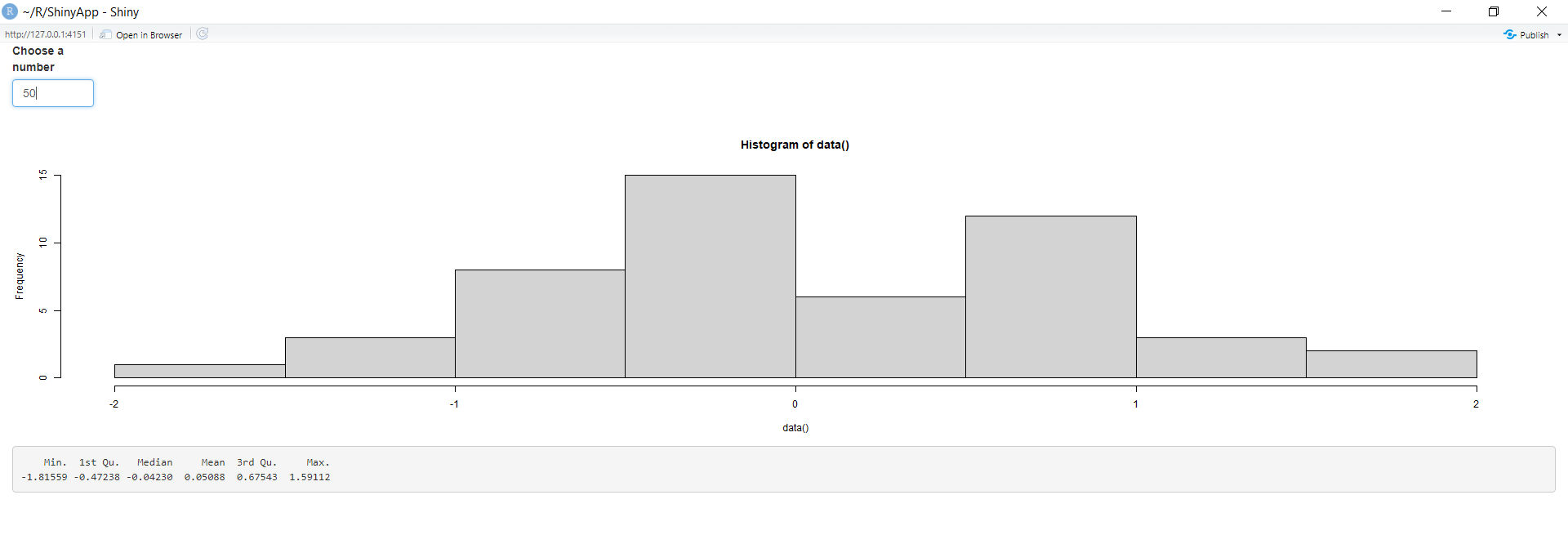Now, change the input value from 50 to 100 to see what happens.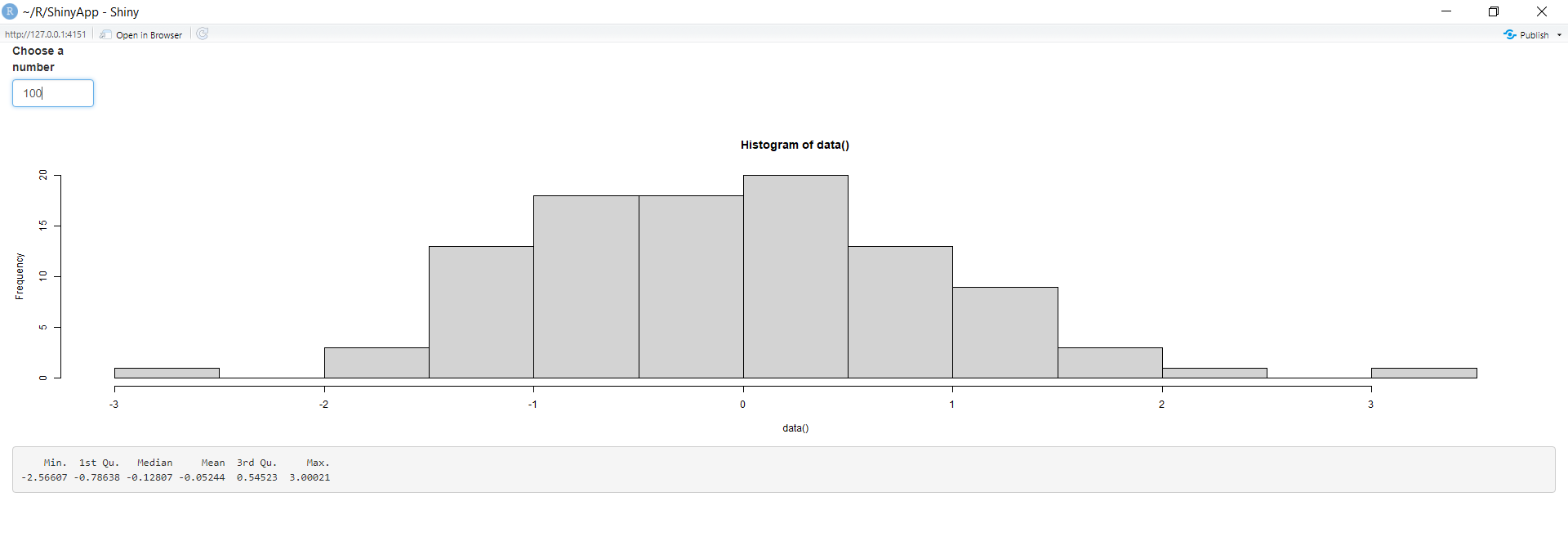The output values(histogram and summary) also change with the alternation in the input values, i.e the app has reactivity.

• observeEvent(): It triggers code to run on servers. Respond to “event-like” reactive inputs, values, and expressions.

Syntax:

observeEvent(eventExpr, handlerExpr, event.env = parent.frame(), event.quoted = FALSE, handler.env = parent.frame(), handler.quoted = FALSE, label = NULL, suspended = FALSE, priority = 0, domain = getDefaultReactiveDomain(), autoDestroy = TRUE, ignoreNULL = TRUE, ignoreInit = FALSE, once = FALSE)

Example:

## R

 `# import shiny package``library``(shiny)` `# define fluid page layout``ui = ``fluidPage``(``  ``textInput``(inputId = ``"num"``,``            ``label = ``"Enter a numeric value"``, value = ``"10"``),``            ``actionButton``(``"button"``, ``"Calculate"``),``  ``column``(8, ``tableOutput``(``"table"``))``)` `server = ``function``(input, output)``{``  ``# Take an action every time button is pressed``  ``observeEvent``(input\$button, {``    ``cat``(``"Showing"``, input\$num, ``"rows\n"``)``  ``})``  ` `  ``# Take a reactive dependency``  ``# on input\$num, but not on any``  ``# of the stuff inside the function``  ``df <- ``eventReactive``(input\$button, {``    ``head``(cars, input\$num)``  ``})``  ``output\$table <- ``renderTable``({``    ``df``()``  ``})``}` `# create shiny app object``# using shinyApp``shinyApp``(ui, server)`

Output: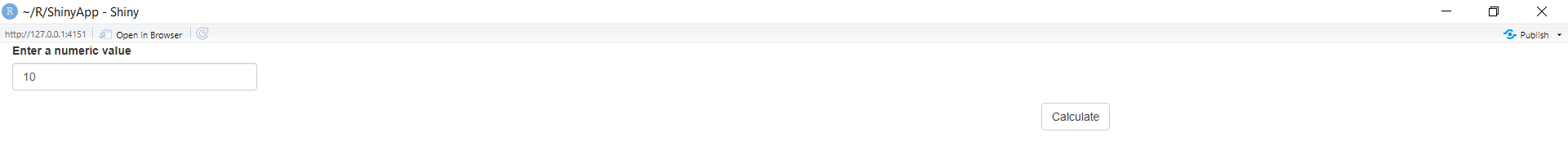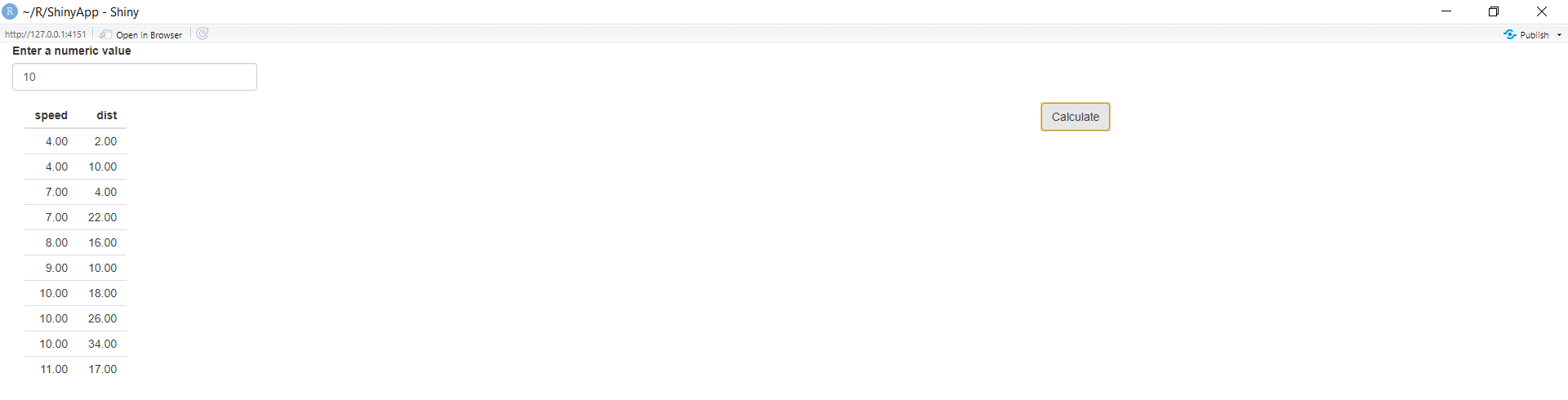• eventReactive(): A reactive expression that only responds to specific values. Respond to “event-like” reactive inputs, values, and expressions.

Syntax:

eventReactive(eventExpr,
valueExpr,
event.env = parent.frame(),
event.quoted = FALSE,
value.env = parent.frame(),
value.quoted = FALSE,
label = NULL,
domain = getDefaultReactiveDomain(),
ignoreNULL = TRUE,
ignoreInit = FALSE)

Example:

## R

 `# import shiny package``library``(shiny)` `# define fluid page layout``ui <- ``fluidPage``(``  ``sliderInput``(inputId = ``"num"``,``              ``label = ``"Choose a number"``,``              ``value = 25, min = 1, max = 100),``  ``actionButton``(inputId = ``"go"``,``               ``label = ``"Update"``),``  ``plotOutput``(``"hist"``)``)` `server <- ``function``(input, output)``{``  ``data <- ``eventReactive``(input\$go, {``    ``rnorm``(input\$num)``  ``})``  ` `  ``output\$hist <- ``renderPlot``({``    ``hist``(``data``())``  ``})``}` `# create shiny app object``# using shinyApp``shinyApp``(ui = ui, server = server)`

Output: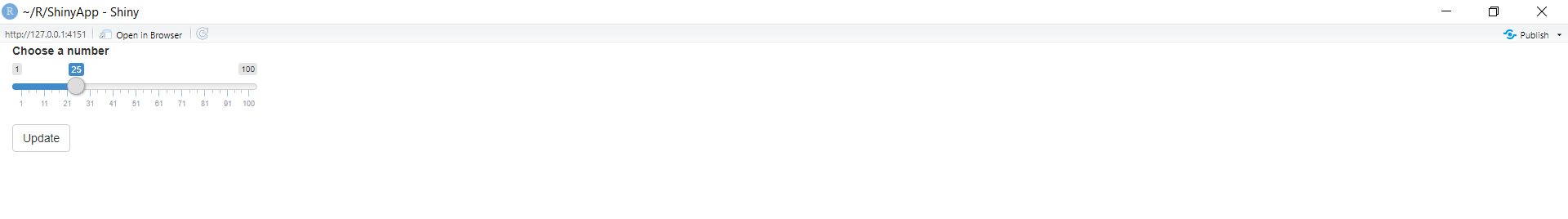Here, the output does not change with the input values until and unless the update button is triggered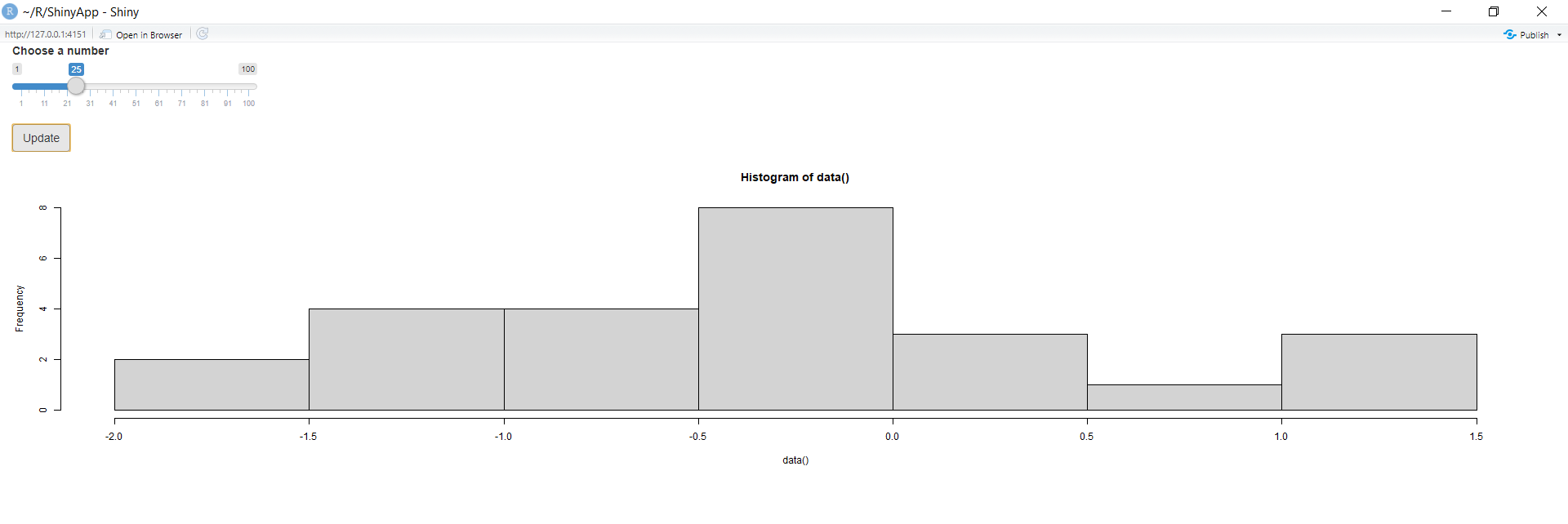• actionButton(): It creates an action button or a link. Their initial value is zero, and increments by one each time it is pressed.

Syntax:

actionButton(inputId, label, icon = NULL, width = NULL, …)

actionLink(inputId, label, icon = NULL, …)

Example:

## R

 `# import shiny package``library``(shiny)` `# define fluid page layout``ui <- ``fluidPage``(``  ``sliderInput``(``"obs"``, ``"Number of observations"``, 0, 1000, 500),``  ``actionButton``(``"goButton"``, ``"Go!"``, class = ``"btn-success"``),``  ``plotOutput``(``"distPlot"``)``)` `server <- ``function``(input, output)``{``  ``output\$distPlot <- ``renderPlot``({``    ``input\$goButton``    ``dist <- ``isolate``(``rnorm``(input\$obs))``    ``hist``(dist)``  ``})``}` `# create shiny app object``# using shinyApp``shinyApp``(ui, server)`

Output: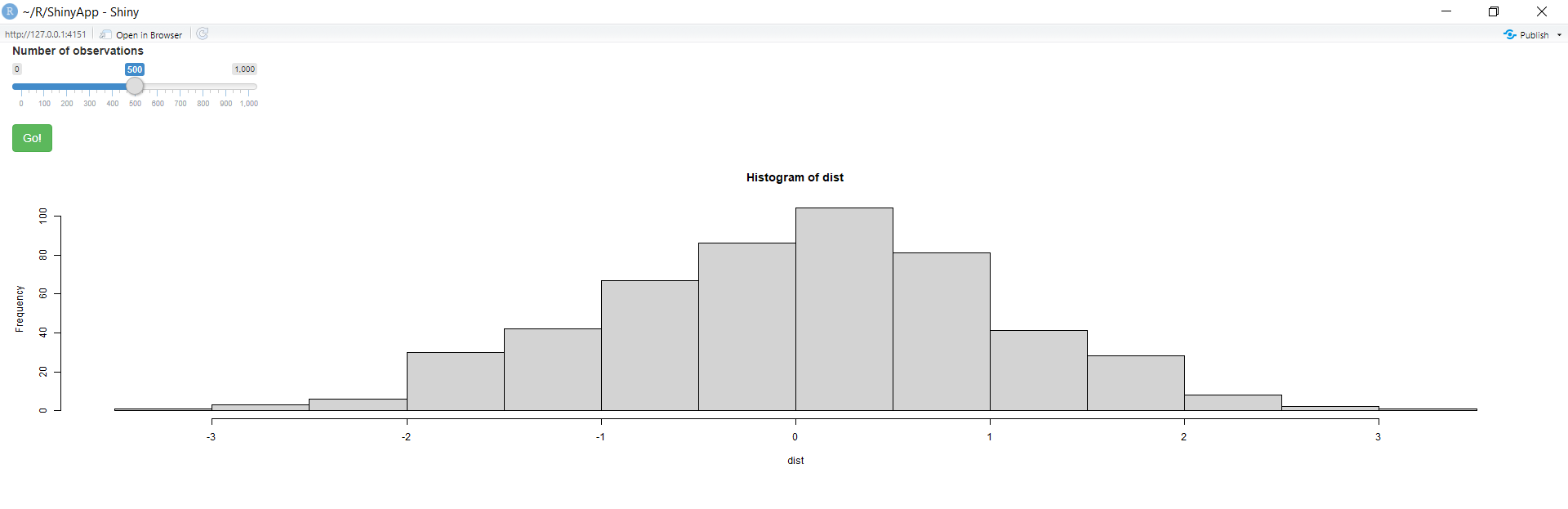The output gets updated as soon as the actionButton(Go!) is clicked.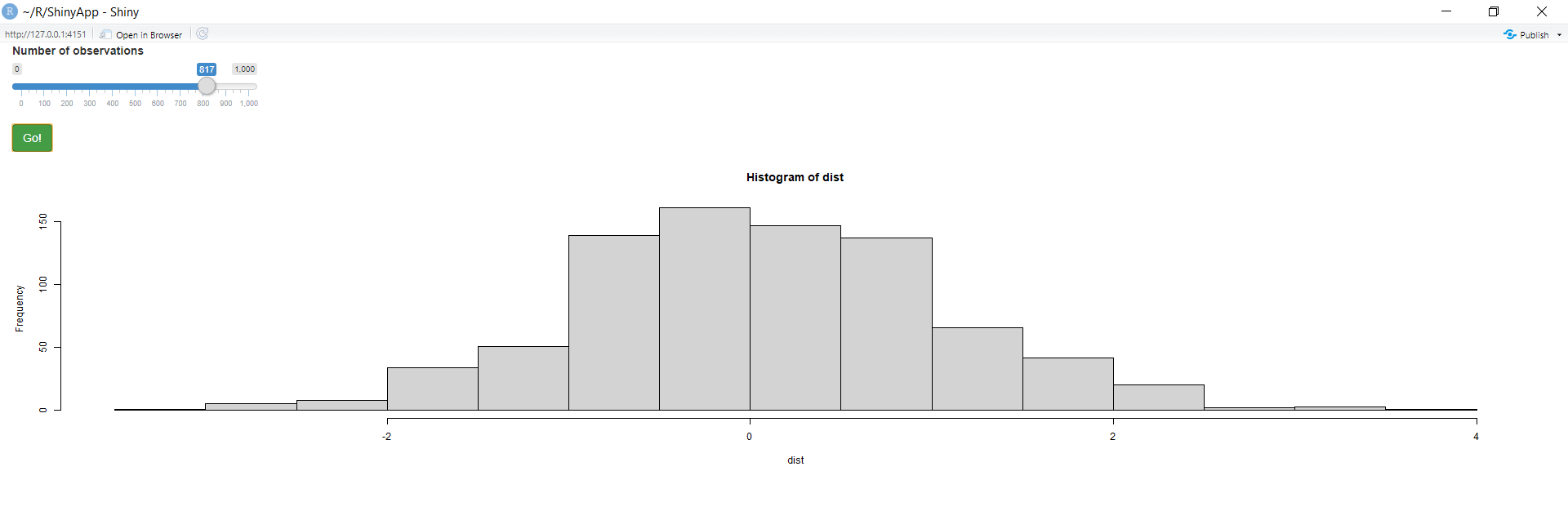• checkboxGroupInput(): It creates a group of checkboxes that can be used to toggle multiple choices independently. The server will receive the input as a character vector of the selected values.

Syntax:

checkboxGroupInput(inputId, label, choices = NULL, selected = NULL, inline = FALSE, width = NULL, choiceNames = NULL, choiceValues = NULL)

Example:

## R

 `# import shiny package``library``(shiny)` `# define fluid page layout``ui <- ``fluidPage``(``  ``checkboxGroupInput``(``"icons"``, ``"Choose icons:"``,``                     ``choiceNames =``                       ``list``(``icon``(``"dog"``), ``icon``(``"cat"``),``                            ``icon``(``"fish"``), ``icon``(``"bug"``)),``                     ``choiceValues =``                       ``list``(``"dog"``, ``"cat"``, ``"fish"``, ``"bug"``)),``                     ``textOutput``(``"txt"``)``)` `server <- ``function``(input, output, session)``{``  ``output\$txt <- ``renderText``({``    ``icons <- ``paste``(input\$icons, collapse = ``", "``)``    ``paste``(``"You chose"``, icons)``  ``})``}` `# create shiny app object``# using shinyApp``shinyApp``(ui = ui, server = server)`

Output: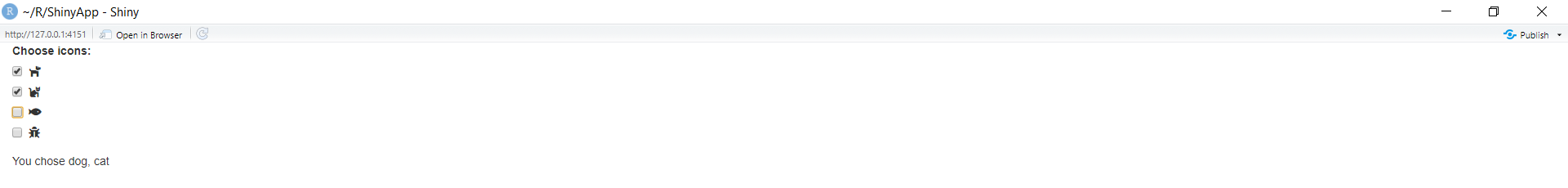• textInput(): It creates a text input label.

Syntax:

textInput(inputId, label, value = “”, width = NULL, placeholder = NULL)

Example:

## R

 `# import shiny package``library``(shiny)` `# define fluid page layout``ui <- ``fluidPage``(``  ``textInput``(``"txt"``, ``"Enter your text here"``, ``"Empty"``),``  ``verbatimTextOutput``(``"value"``)``)` `server <- ``function``(input, output)``{``  ``output\$value <- ``renderText``({ input\$text })``}` `# create shiny app object``# using shinyApp``shinyApp``(ui, server)`

Output: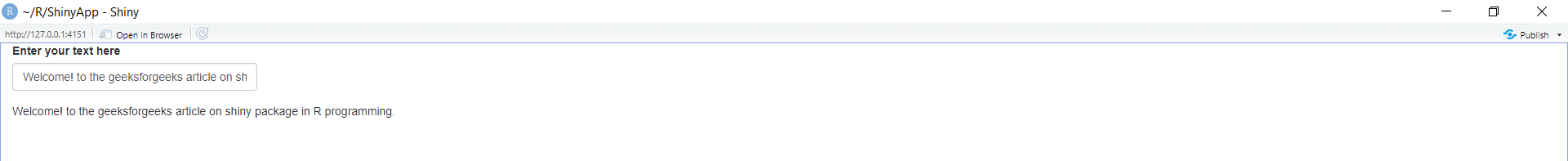• textOutput(): It creates a text output element. Render a reactive output variable as text within an application page.

Syntax:

textOutput(outputId, container = if (inline) span else div, inline = FALSE)

Example:

## R

 `# import shiny package``library``(shiny)` `# define fluid page layout``ui <- ``fluidPage``(`` ``textInput``(inputId = ``"Name"``, label = ``"Enter your name"``),`` ``textOutput``(``"txt"``)``)` `server <- ``function``(input, output, session)``{``  ``output\$txt <- ``renderText``({``    ``Name <- ``paste``(input\$Name, collapse = ``", "``)``    ``paste``(``"Welcome! to geeksforgeeks "``, Name)``  ``})``}` `# create shiny app object``# using shinyApp``shinyApp``(ui = ui, server = server)`

Output: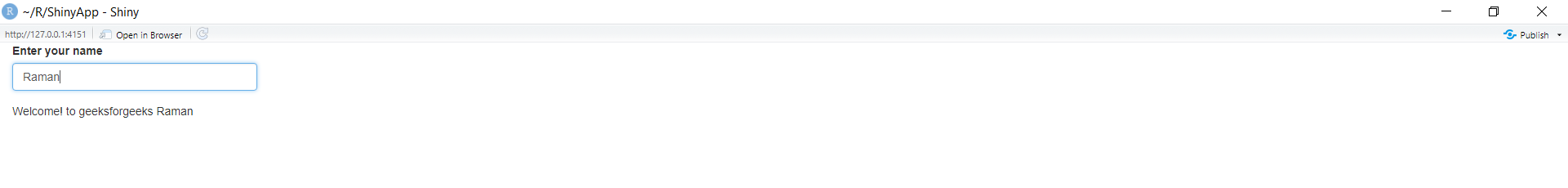• wellPanel(): Creates a panel with a slightly inset border and gray background.

Syntax: wellPanel(…)

Example:

## R

 `# import shiny package``library``(shiny)` `# define fluid page layout``ui <- ``fluidPage``(``    ``sliderInput``(inputId = ``"num"``,``                ``label = ``"Choose a number"``,``                ``value = 25, min = 1, max = 100),``  ` ` ``# define plot inside`` ``# a wellPanel`` ``wellPanel``(``plotOutput``(``"hist"``))``)` `server <- ``function``(input, output)``{``  ``output\$hist <- ``renderPlot``({``    ``hist``(``rnorm``(input\$num), main = input\$title)``  ``})``}` `# create shiny app object``# using shinyApp``shinyApp``(ui = ui, server = server)`

Output: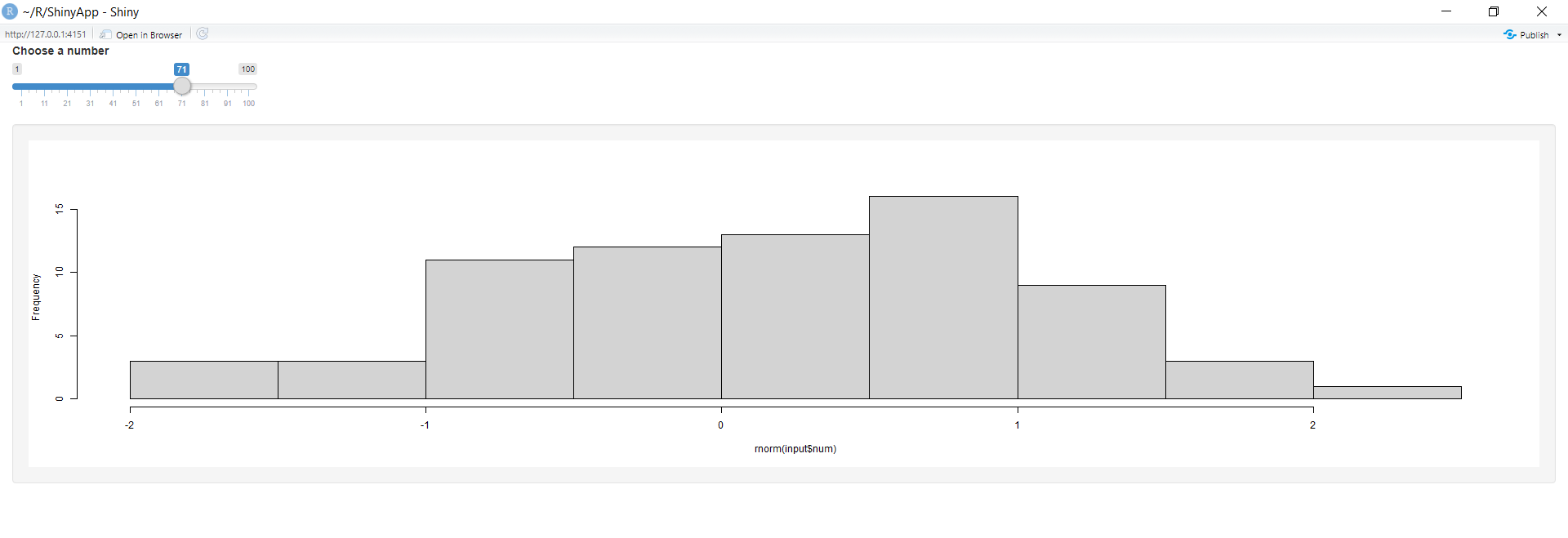Observe that the histogram lies inside a gray-colored box(wellPanel).

My Personal Notes arrow_drop_up